# 寻找丢失的数据与预测未来_中小学生的线性回归方程与Python### Python海龟宝典含200多个原创的用turtle模块制作的创意程序，原名《Python趣味编程200例》。准备参加全国创意编程与智能设计大赛的同学们可以用来做参考。

```x_list = [2015,2019,2020]
y_list = [10.2,18.1,20.3]

n = len(x_list)
xmeans = sum(x_list)/n
ymeans = sum(y_list)/n

f1 = sum([x*y for x,y in zip(x_list,y_list)])
f2 = n * xmeans * ymeans
f3 = sum([x*x for x in x_list])
f4 = n * xmeans * xmeans
b = (f1-f2)/(f3-f4)
a = ymeans - b * xmeans

# 请把下面的代码用for循环实现
y2016 = round(b * 2016 + a,2)
print('2016年的税收金额大概是:',y2016)

y2017 = round(b * 2017 + a,2)
print('2017年的税收金额大概是:',y2017)

y2018 = round(b * 2018 + a,2)
print('2018年的税收金额大概是:',y2018)

y2021 = round(b * 2021 + a,2)
print('2021年的税收金额大概是:',y2021)

y2022 = round(b * 2022 + a,2)
print('2022年的税收金额大概是:',y2022)

y2023 = round(b * 2023 + a,2)
print('2023年的税收金额大概是:',y2023)

y2024 = round(b * 2024 + a,2)
print('2024年的税收金额大概是:',y2024)

```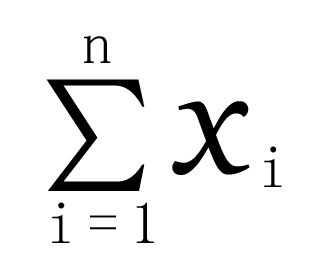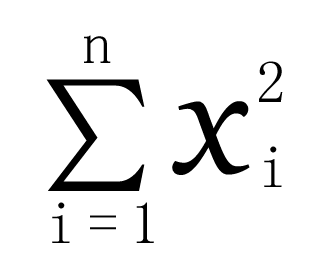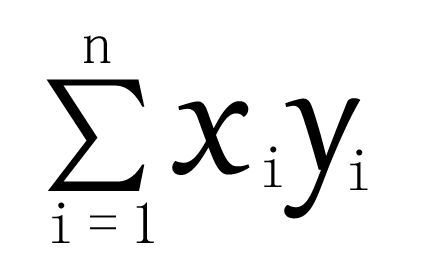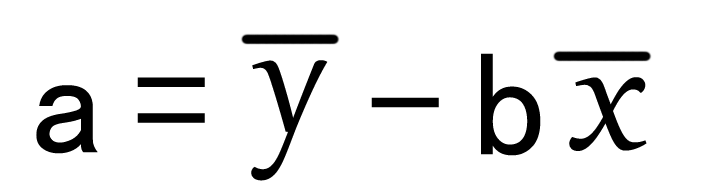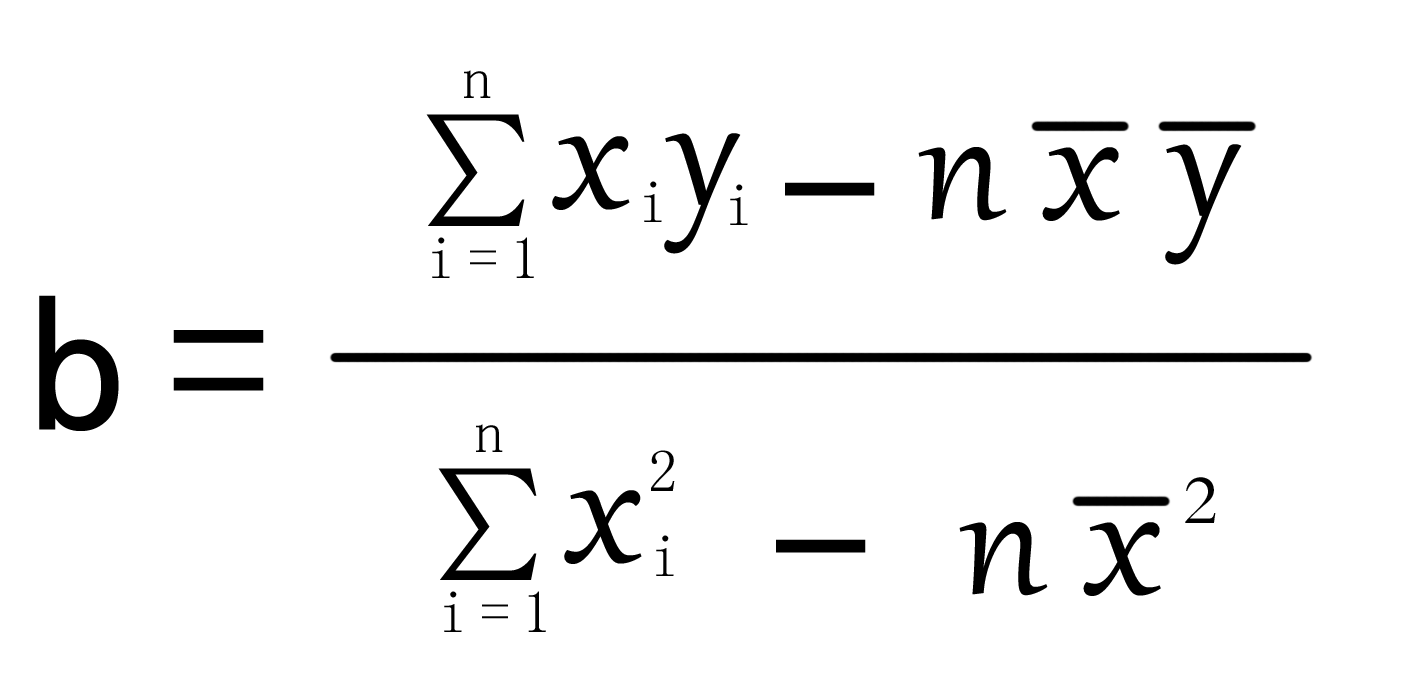#### 学本领，探索更大的世界！

error: Content is protected !!